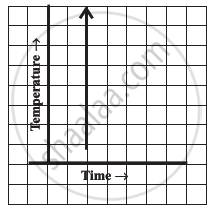Share

# Can There Be a Time-temperature Graph as Follows? Justify Your Answer - 3 - CBSE Class 8 - Mathematics

ConceptConcept of a Line Graph

#### Question#### Solution

This cannot be a time−temperature graph since different temperatures at the same time are not possible

Is there an error in this question or solution?

#### APPEARS IN

NCERT Solution for Mathematics Textbook for Class 8 (2018 to Current)
Chapter 15: Introduction to Graphs
Ex. 15.10 | Q: 7.3 | Page no. 239
Solution Can There Be a Time-temperature Graph as Follows? Justify Your Answer - 3 Concept: Concept of a Line Graph.
S